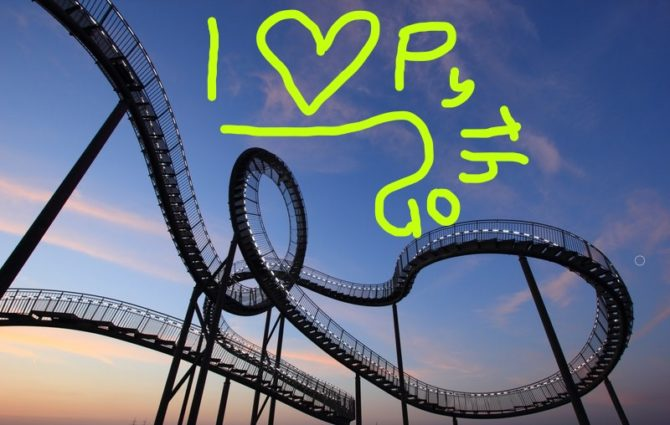# Python Puzzle Rush: Looping Louis🐍

This article gives you five code puzzles to help you master basic looping in Python.

• If you are a Python expert, you solve all of them without even the slightest mistake.
• If you are a Python intermediate, you make one or two mistakes.
• If you are a Python beginner, you should get at least one right.

Have a look at your clock: 5-minutes starting now…. Go, go, go!

## Puzzle 1

```n = 16
x = 0

while x < 3:
n /= 2
x += 1

print(n)```

What’s the output of this code snippet?

## Puzzle 2

```inc = lambda x : x + 1

print(inc(2))```

What’s the output of this code snippet?

## Puzzle 3

```index = 5
string = 'g'

while index > 3:
index -= 1
string += 'o'
else:
string += 'd'

print(string)```

What’s the output of this code snippet?

## Puzzle 4

```index = 5
string = 'g'

while index > 3:
index -= 1
string += 'o'
if index == 3:
break
else:
string += 'd'

print(string)```

What’s the output of this code snippet?

## Puzzle 5

```index = 5
string = 'g'

while index > 3:
index -= 1
if index == 3:
continue
string += 'o'

else:
string += 'd'

print(string)```

What’s the output of this code snippet?

## Solutions

How many puzzles have you solved correctly? Here are the correct solutions:

• Puzzle 1: 2.0
• Puzzle 2: 3
• Puzzle 3: good
• Puzzle 4: goo
• Puzzle 5: god

If you have at least four correct puzzles, you already belong to the top 20% of Python coders.

## Background

Need a bit of Python background to understand the puzzles? Watch my short explainer video.

How many puzzles have you solved correctly? Comment your rating and experience below! 🐍🤓👌